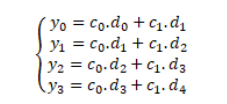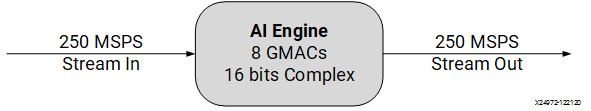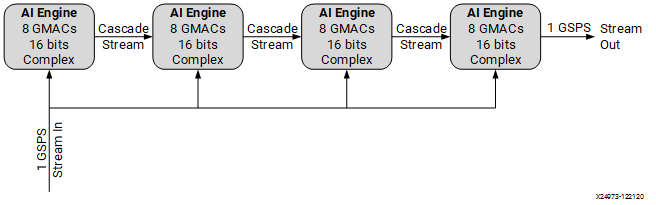# 1 Gsps Implementation with Cascade Stream - 2022.1 English

## AI Engine Kernel Coding Best Practices Guide (UG1079)

Document ID
UG1079
Release Date
2022-05-25
Version
2022.1 English

The AI Engine vector unit supports 8 MACs per cycle for cint16 multiply-accumulate cint16 types. If a four lane implementation of mul4/mac4 intrinsics is adopted, then there will be two complex operations on each lane.16 mac4() are needed for computing four outputs because each output requires 32 complex MACs. This means, to compute four outputs, 16 cycles using an AI Engine are required. So the sample rate of an AI Engine (assuming it runs at 1 GHz) would be as follows.

4 Gsps/16 = 0.25 Gsps = 250 Msps

This calculates the compute bound of an AI Engine. However, the memory bound to see if that sample rate can be met still needs to be considered. Assume that only one stream input and one stream output are used for data transfer and the coefficients are stored in the AI Engine internal memory. The stream interface of an AI Engine supports 32 bits per cycle. It is capable of transferring one sample of data every cycle. Thus, the sample rate from the data transferring view is as follows.

1 sample/cycle *1 GHz = 1 Gsps

It is larger than compute bound, which is 250 Msps. So an AI Engine implementation will operate at 250 Msps.

Figure 1. One AI Engine FIR Filter RealizationBased on the calculations, it is possible to achieve 1 Gsps via a stream input and output stream interface. If the MAC operations of a single kernel implementation are split into four kernels, 4*250Msps = 1 Gsps, compute throughput can be achieved. Those four kernels are connected through cascade streaming. Therefore, the AI Engine compute bound matches AI Engine interface throughput.

Figure 2. 1 Gsps Implementation with Four Cascaded Kernels